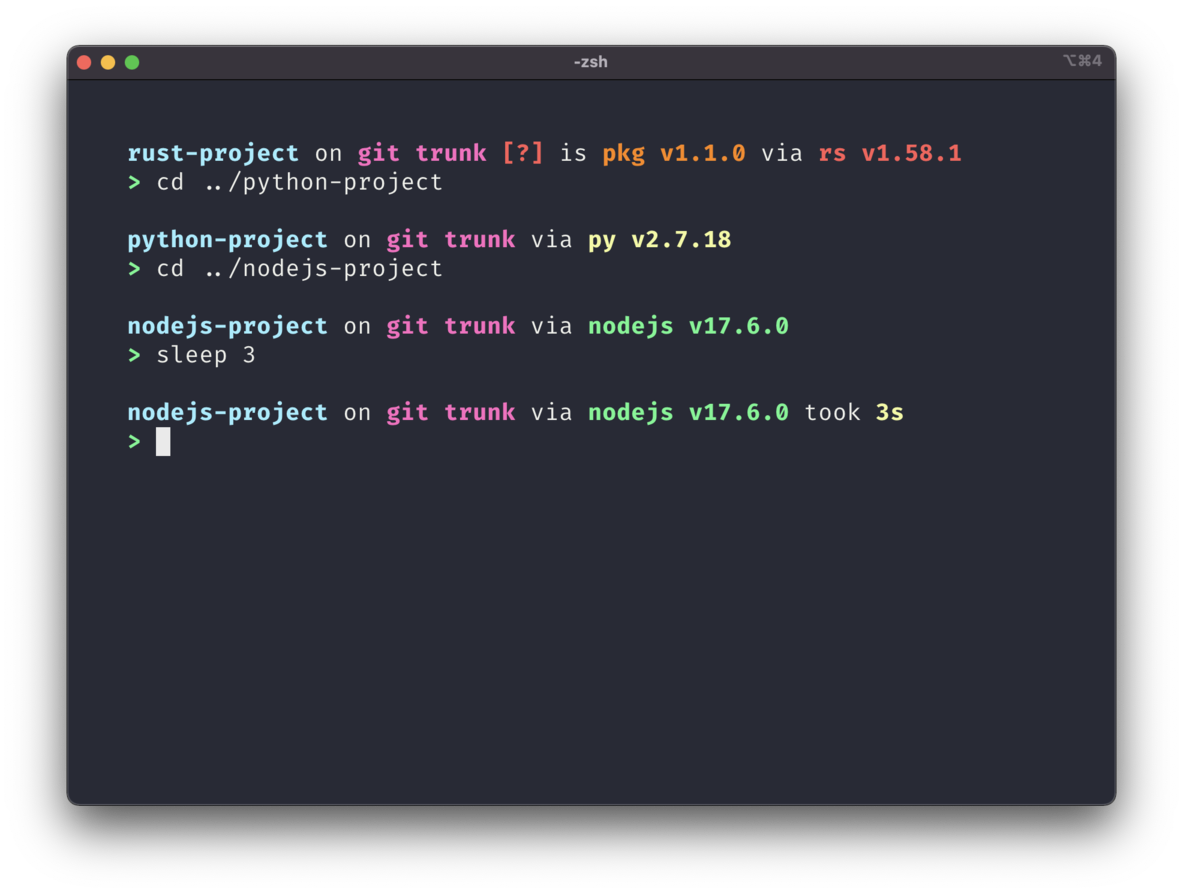## # Plain Text Symbols Preset

This preset changes the symbols for each module into plain text. Great if you don't have access to Unicode.### # Configuration

``````starship preset plain-text-symbols -o ~/.config/starship.toml
``````

``````[character]
success_symbol = "[>](bold green)"
error_symbol = "[x](bold red)"
vimcmd_symbol = "[<](bold green)"

[git_commit]
tag_symbol = " tag "

[git_status]
behind = "<"
diverged = "<>"
renamed = "r"
deleted = "x"

[aws]
symbol = "aws "

[azure]
symbol = "az "

[bun]
symbol = "bun "

[c]
symbol = "C "

[cobol]
symbol = "cobol "

[conda]
symbol = "conda "

[crystal]
symbol = "cr "

[cmake]
symbol = "cmake "

[daml]
symbol = "daml "

[dart]
symbol = "dart "

[deno]
symbol = "deno "

[dotnet]
symbol = ".NET "

[directory]

[docker_context]
symbol = "docker "

[elixir]
symbol = "exs "

[elm]
symbol = "elm "

[fennel]
symbol = "fnl "

[fossil_branch]
symbol = "fossil "

[gcloud]
symbol = "gcp "

[git_branch]
symbol = "git "

[golang]
symbol = "go "

[guix_shell]
symbol = "guix "

[hg_branch]
symbol = "hg "

[java]
symbol = "java "

[julia]
symbol = "jl "

[kotlin]
symbol = "kt "

[lua]
symbol = "lua "

[nodejs]
symbol = "nodejs "

[memory_usage]
symbol = "memory "

[meson]
symbol = "meson "

[nim]
symbol = "nim "

[nix_shell]
symbol = "nix "

[ocaml]
symbol = "ml "

[opa]
symbol = "opa "

[os.symbols]
Alpaquita = "alq "
Alpine = "alp "
Amazon = "amz "
Android = "andr "
Arch = "rch "
Artix = "atx "
CentOS = "cent "
Debian = "deb "
DragonFly = "dfbsd "
Emscripten = "emsc "
EndeavourOS = "ndev "
Fedora = "fed "
FreeBSD = "fbsd "
Garuda = "garu "
Gentoo = "gent "
HardenedBSD = "hbsd "
Illumos = "lum "
Linux = "lnx "
Mabox = "mbox "
Macos = "mac "
Manjaro = "mjo "
Mariner = "mrn "
MidnightBSD = "mid "
Mint = "mint "
NetBSD = "nbsd "
NixOS = "nix "
OpenBSD = "obsd "
OpenCloudOS = "ocos "
openEuler = "oeul "
openSUSE = "osuse "
OracleLinux = "orac "
Pop = "pop "
Raspbian = "rasp "
Redhat = "rhl "
RedHatEnterprise = "rhel "
Redox = "redox "
Solus = "sol "
SUSE = "suse "
Ubuntu = "ubnt "
Unknown = "unk "
Windows = "win "

[package]
symbol = "pkg "

[perl]
symbol = "pl "

[php]
symbol = "php "

[pijul_channel]
symbol = "pijul "

[pulumi]
symbol = "pulumi "

[purescript]
symbol = "purs "

[python]
symbol = "py "

[raku]
symbol = "raku "

[ruby]
symbol = "rb "

[rust]
symbol = "rs "

[scala]
symbol = "scala "

[spack]
symbol = "spack "

[solidity]
symbol = "solidity "

[status]
symbol = "[x](bold red) "

[sudo]
symbol = "sudo "

[swift]
symbol = "swift "

[terraform]
symbol = "terraform "

[zig]
symbol = "zig "
``````